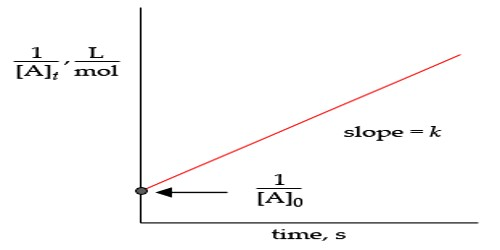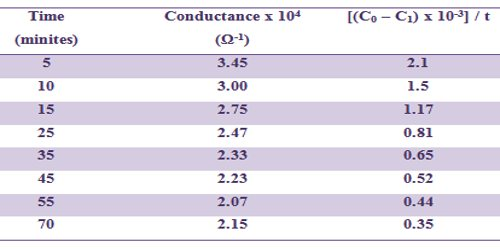Chemistry

# Second Order Reaction: Hydrolysis of Several EstersSecond Order Reaction: Hydrolysis of Several Esters

Hydrolysis of several esters of the aliphatic series in alkaline aqueous medium obey strictly second order law. (Note that hydrolysis of esters in acid medium is kinetically of the first- order). Ethyl acetate is hydrolysed as follows:

CH3COOC2H5 (aq) + NaOH (aq) → CH3COONa (aq) + C2H5OH (aq).

The acetic acid formed as a result of hydrolysis instantaneously reacts with NaOH to form sodium acetate. The reaction can be followed by measuring quantitatively the amount of NaOH left unreached by titrating against a standard acid solution. A more convenient method of following this reaction is to measure the electrical conductance as a function of time. Since as a result of the reaction highly mobile hydroxyl ions are replaced by less mobile acetate ions, the conductance falls as the reaction proceeds. If the initial concentration, of NaOH and ethyl acetate are the same. Introducing the conductance in the rate expression when C1 and C0 are respectively the conductance at time t and at zero times and a is the initial concentration one can see that –

Ct = 1 /ak . (C0 – C1)/t + C0

A plot of C1 vs (C0 – C1)/t would yield a straight line of slope = 1/ak (figure)Fig: A plot of C1 vs (C0 – C1)/t

In table such data are included and a linear plot according to above equation can be obtained.

Table: Hydrolysis of ethyl acetate by NaOH at 320C

[NaOH] = 0.01 mol L-1 and [EtAc] = 0.01 mol L-1K = 12.7 L mol-1 min-1